## Cubes and Dices

#### Cubes and Dices

1. Which colour is opposite to Green?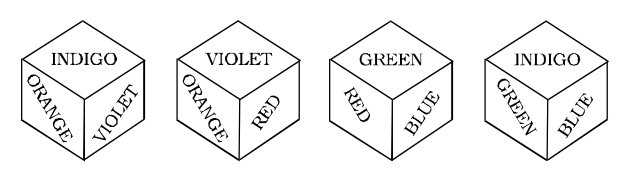1. On the basis of question figure pattern ,
The six colours are: Indigo, Violet, Orange, Red, Green and Blue.
The colours Indigo, Blue and Red are on the faces adjacent to Green and therefore, these colours cannot be on the face opposite to Green.

##### Correct Option: D

On the basis of question figure pattern ,
The six colours are: Indigo, Violet, Orange, Red, Green and Blue.
The colours Indigo, Blue and Red are on the faces adjacent to Green and therefore, these colours cannot be on the face opposite to Green.
After careful observation of all the views of dice, it is clear that Orange lies opposite to Green.

1. Three positions of a cube are given. Based on them find out which number is found opposite of number 2 in a given cube?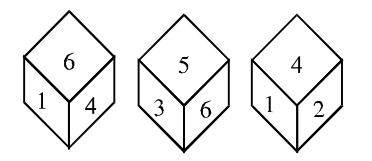1. As per the given pattern in question figure , we can find the similar next position of given dice .
The numbers b>1, 3, 4 and 5 are on adjacent faces of the number

##### Correct Option: D

As per the given pattern in question figure , we can find the similar next position of given dice .
The numbers 1, 3, 4 and 5 are on adjacent faces of the number
Therefore, 6 lies opposite 2.

1. Study the two different positions of a dice. When the face containing one dot is at bottom then how many dots would be there on the top face?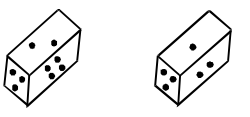1. As per the given pattern in question figure , we can find the similar next position of given dice .
Here 2 and 3 dots are at the same side .

##### Correct Option: C

As per the given pattern in question figure , we can find the similar next position of given dice .
When both 2 and 3 dots are at the same side, 1 dot should be opposite to 4 dot.

1. Two positions of a dice are shown below. When 3 is at the bottom, which number is at the top?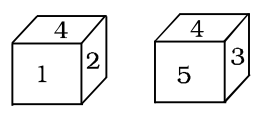1. As we can see that ,
In these two positions one common face with number 4 is in the same position.

##### Correct Option: C

As we can see that ,
In these two positions one common face with number 4 is in the same position.
According to the rule number (3) of dice ,we get
1 lies opposite 5.
2 lies opposite 3.
4 lies opposite 6.

1. Two positions of a dice are shown below. When 4 is at the bottom, what will be at the top?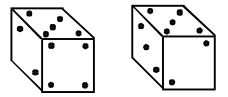1. On the basis of following pattern in given dices , we can say that
Here 5 and 2 dots are at the same side .

##### Correct Option: B

On the basis of following pattern in given dices , we can say that
Here 5 and 2 dots are at the same side .
From the two views of the dice, it is clear that when 4 is at the bottom, 3 will be on the top.